# Arithmetic and geometric

## Determination of average values

### The arithmetic mean

Definition: the arithmetic mean of several numbers is a number, the sum of these numbers divided by their number.

To calculate the value of the average value, you must add values measured in the same units.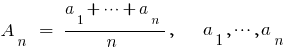— any number

Is it possible to find average of three, four or more numbers? So.

3адача 1. To find average of numbers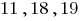Solution: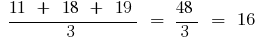3адача 2. To find the arithmetic mean of four numbers, if their sum is equal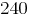.

Solution: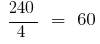The arithmetic mean is used to calculate the average.

### The challenge for the arithmetic mean

While traveling in the car for the first hour we drove 150 km in the second hour to 190 km, and for the third and fourth — at 80 km. the average number per hour drove the car?

Solution: In this problem you need to find the arithmetic mean of the numbers 190, 150, 80, 80.— average per hour drove the car

### The geometric mean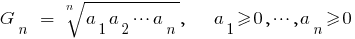### Harmonic mean of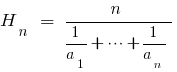### Mean square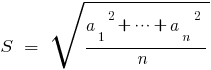## The overall ratio between the average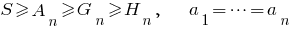## The Cauchy Inequality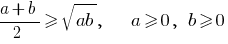## Evaluation of the sum of two mutually inverse numbers

If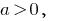that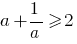If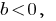that## Evaluation of sums of the squares of three numbers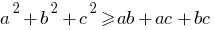## Methods of proof of inequalities

1. The compilation of the difference between the left and right side (if the difference is positive, the left side is greater than right)
2. The use of special vanich inequalities and properties of the numerical inequalities, in particular inequalities with the use of transitional
3. The use of growth or espadana functions and use the derivative
Tags:
Chapter:
Versions in other languages:
Share with friends: